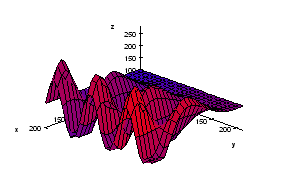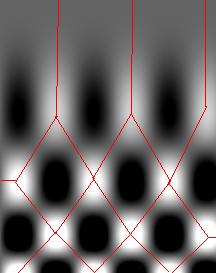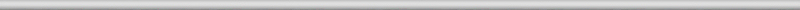## August 24, 2008

### Watershed image segmentation, part 1

Previously we discussed the watershed algorithm for binary images. One thing that wasn’t explained was where the name comes from.

We start with the following approach. According to Gonzales and Woods: “we think of a gray scale image as a topological surface, where the values of f(x,y) are interpreted as heights.” This is good (except the redundant “topological”) and quite clear. Mathematically, if f(x,y) gives the value of gray of the pixel (x,y), we simply end up with the -graph of f (remember precalc?).Next, we find the “catchment” basins. Mathematically, these are minimum points of the surface. However, to find basins’ borders we need to find the ridge lines that separate them. Mathematically, those are lines that go from one maximum point to another via the saddle points.

To summarize, we create a surface from the image by using the value of gray at a given pixel as the height of the surface above it. The light areas are the peaks and the dark areas are the valleys. Next, we flood the valleys, gradually. As we do that, we don’t allow the water to flow from one valley to another. How? By building dams. These dams will break the image into regions each containing a single valley. That’s image segmentation.

Let’s now take a look at the Wikipedia article: “The watershed algorithm is an image processing segmentation algorithm that splits an image into areas, based on the topology of the image.” First, any segmentation algorithm splits an image into areas. Second, any segmentation should be based on the topology of the image. So, what’s left is “The watershed is an image segmentation algorithm”.

The next sentence is “The length of the gradients is interpreted as elevation information.” Wait a minute, that’s not the same! The length of the gradient is the steepness of the surface. In the next sentence however the article seems comes back to the standard approach: “During the successive flooding of the grey value relief, watersheds with adjacent catchment basins are constructed.” And then again: “This flooding process is performed on the gradient image…” Using the gradient as the surface is an alternative approach to the watershed, so this must be a mix-up. Another approach is using the distance function for binary images.

We’ll discuss these issues in the next post.